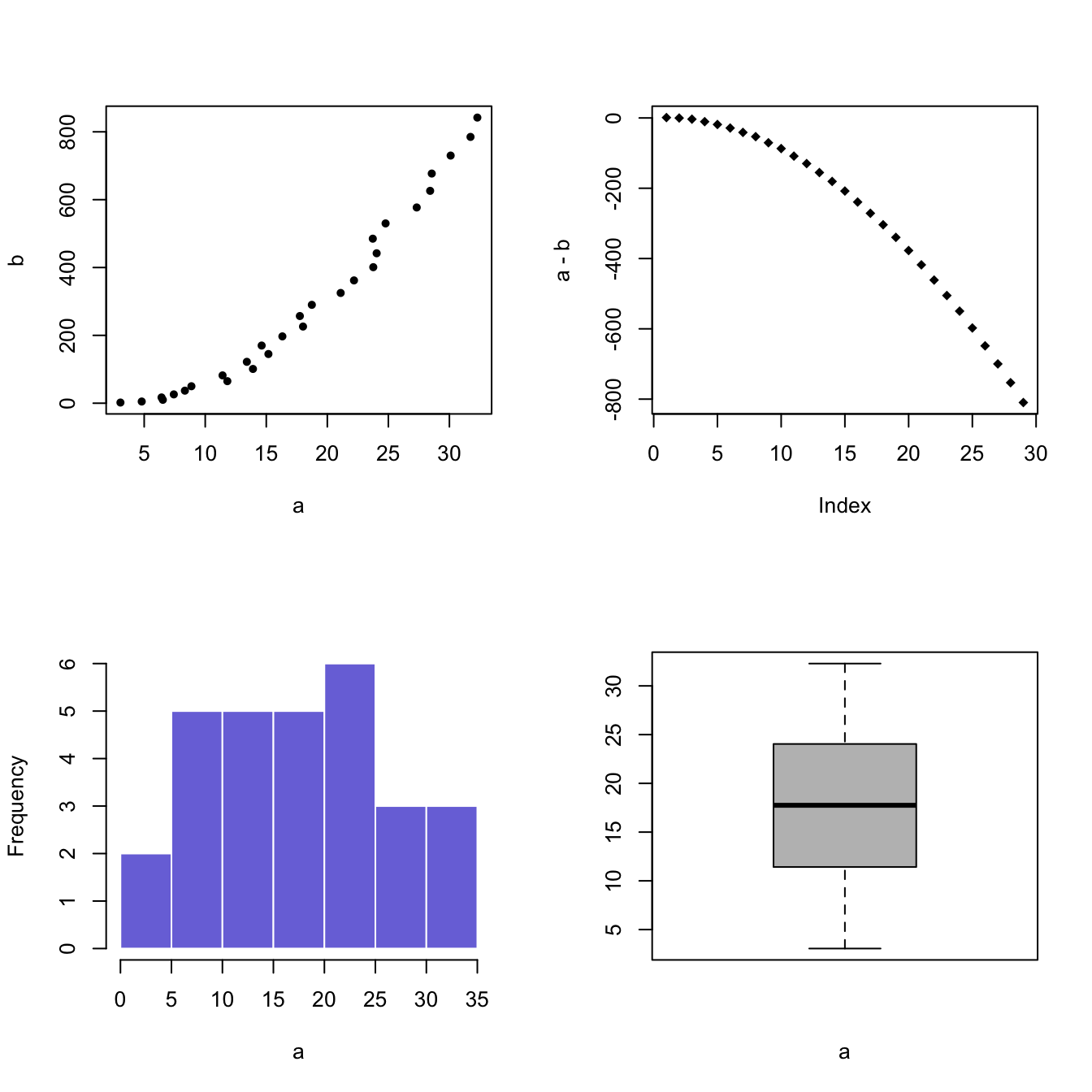# Use par mfrow to split screen

The `par()` function allows to set the `mfrow()` parameters to cut the charting window in several section.

# Most basic scatterplot

The `par()` function allows to set parameters to the plot. The `mfrow()` parameter allows to split the screen in several panels. Subsequent charts will be drawn in panels.

You have to provide a vector of length 2 to `mfrow()`: number of rows and number of columns.

Note: `mfcol()` does the same job but draws figure by columns instead of by row.

Alternative: see the layout() function for more complex layout creation.``````#Create data
a <- seq(1,29)+4*runif(29,0.4)
b <- seq(1,29)^2+runif(29,0.98)

#Divide the screen in 2 columns and 2 lines
par(mfrow=c(2,2))

#Add a plot in each sub-screen !
plot( a,b , pch=20)
plot(a-b , pch=18)
hist(a, border=F , col=rgb(0.2,0.2,0.8,0.7) , main="")
boxplot(a , col="grey" , xlab="a")``````

Related chart types

## Contact

This document is a work by Yan Holtz. Any feedback is highly encouraged. You can fill an issue on Github, drop me a message on Twitter, or send an email pasting yan.holtz.data with gmail.com.